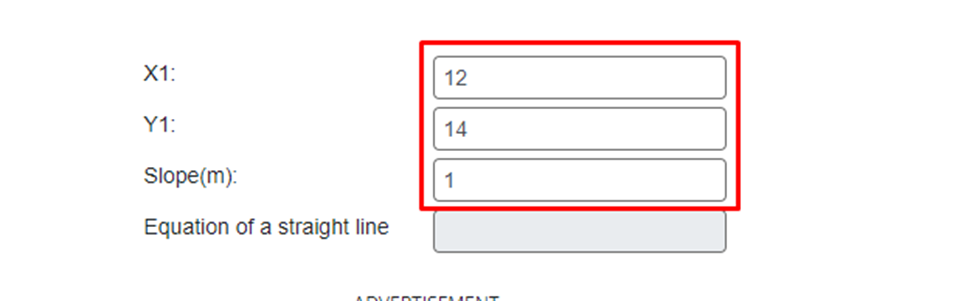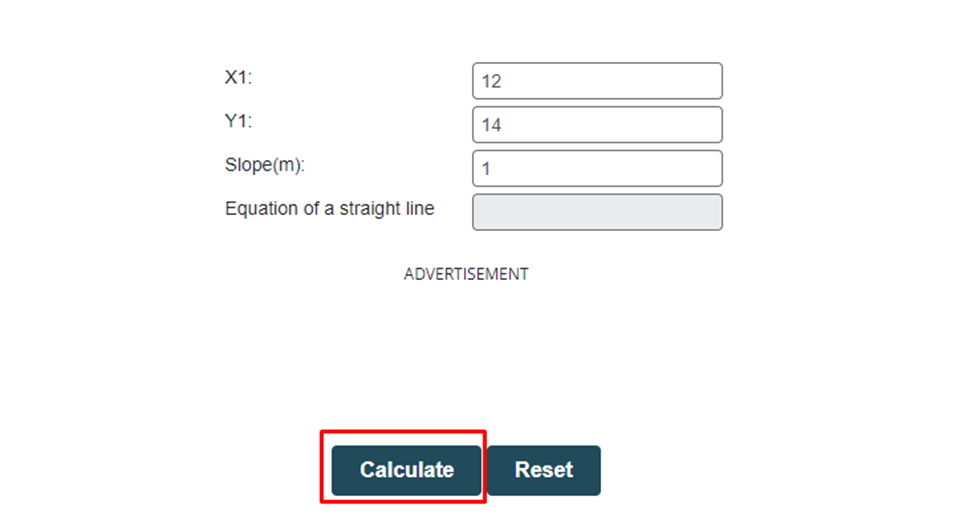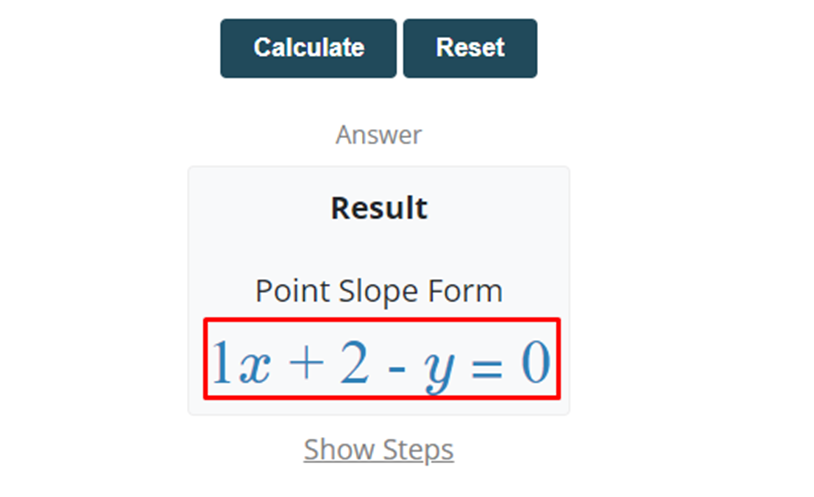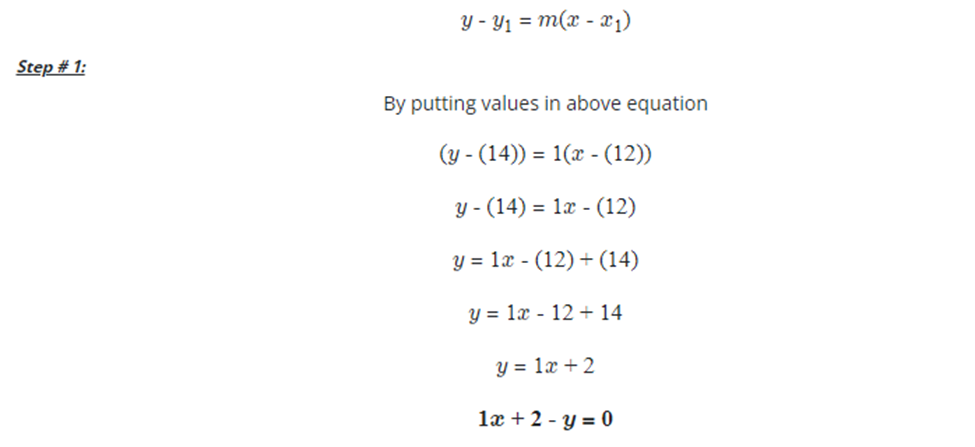## What is the point-slope form and how to use it to find the equation of the line?

In algebra, the point-slope form is frequently used to find the linear equation of the line by using the slope of the line. The equation of the line is used in various forms of differential equations. It is usually known as the linear equation because it has variables of degree one.

There are various methods in algebra to determine the equation of the line. The methods are the point-slope form, slope-intercept form, and intercept form. In this post, will learn about the equation of the line, the point-slope form, and how to calculate the line’s equation by using it.

## What is the point-slope form?

The point-slope form is a general technique or method used to find the equation of the line. According to the name of the point-slope, this method uses the slope of the line and a pair of the number of the line.

The slope of the line can be used by using various methods like the two points method or the equation of the line. But we have to calculate the equation of the line so we can use the two points method to determine the slope of the line.

The slope is generally the fraction of change in the value of y and the change in the values of x. in simple words, the slope of the line is the rise over run of the given points. The points of the line may be positive or negative integers.

Slope of the line = rise / run

Slope of the line = y2 – y1 / x2 – x1

The slope of the line can also be calculated with steps by using a slope calculator.

In the point-slope form, if the slope is not given you can easily calculate it by using the formula of the slope of the line. After determining the slope of the line takes a pair of points of the line. Then substitute the slope of the line and pair of the points in the equation of the point-slope.

After simplifying the equation of the point-slope form you’ll get the equation of the line. The equation of the point-slope form is given below.

y – y1 = m (x – x),

·         x & y are the fixed variables of the linear equation of the line.

·         x1 & yare the pair of the points of the line.

·         m is the slope of the line.

## How to calculate the line’s equation by using the point-slope form?

By using the general equation of the point-slope form, you can easily find the linear equation of the line. You must be familiar with the slope of the line to calculate the equation of the line. Follow the below steps to calculate the equation of the line.

·         First of all, find the slope of the line, if it is not given.

·         Take the pair of points.

·         Write the general equation of the point-slope form.

·         Put the values in the formula of the point-slope form.

·         The result will be the linear equation of the line.

Let us take some examples to learn how to calculate the linear equation of the line.

Example 1: For positive integers

Find the linear equation of the line by using the point-slope form of the given pairs of numbers, (12, 14) and (22, 24).

Solution

Step 1: First of all, take the given pairs of numbers and give names to each point.

x1 = 12, x2 = 22, y1 = 14, y2 = 24

Step 2: Write the general formula of the slope of the line.

Slope of the line = y2 – y1 / x2 – x1

Step 3: Put the given pairs of numbers in the formula of the slope.

Slope = m = (y2 – y1) / (x2 – x1)

Slope = m = (24 – 14) / (22 – 12)

Slope = m = (10) / (10)

Slope = m = 10 / 10

Slope = m = 1/1

Slope = m = 1

Step 4: Write the general formula of the point-slope form.

y – y1 = m (x – x)

Step 5: Put any pair of numbers and calculated slope of the line in the formula of the point-slope form to get the linear equation of the line.

y – y1 = m (x – x)

y – (14) = 1(x – 12)

y – 14 = x – 12

y – 14 – x + 12 = 0

y – x – 2 = 0

x – y + 2 = 0

To avoid the large number of steps to calculate the linear equation of the line, you can use a point slope form calculator. Follow the below steps to calculate the equation of the line according to the formula of the point-slope form.

Step 1: Input the pair of points and the slope of the line.Step 2: Press the calculate button.Step 3: The result will show below the calculate button.Step 4: Press the show more button to view the solution with steps.Example 1: For positive integers

Find the linear equation of the line by using the point-slope form of the given pairs of numbers, (-2, -5) and (-4, -15).

Solution

Step 1: First of all, take the given pairs of numbers and give names to each point.

x1 = -2, x2 = -4, y1 = -5, y2 = -15

Step 2: Write the general formula of the slope of the line.

Slope of the line = y2 – y1 / x2 – x1

Step 3: Put the given pairs of numbers in the formula of the slope.

Slope = m = (y2 – y1) / (x2 – x1)

Slope = m = (-15 – (-4)) / (-4 – (-2))

Slope = m = (-15 + 4) / (-4 + 2)

Slope = m = (-11) / (-2)

Slope = m = -11 / -2

Slope = m = 11/2

Slope = m = 5.5

Step 4: Write the general formula of the point-slope form.

y – y1 = m (x – x)

Step 5: Put any pair of numbers and calculated slope of the line in the formula of the point-slope form to get the linear equation of the line.

y – y1 = m (x – x)

y – (-4) = 5.5(x – 2)

y + 4 = 5.5x – 11

y + 4 – 5.5x + 11 = 0

y – 5.5x + 15 = 0

5.5x – y – 15 = 0

## Summary

The point-slope form is a well-known method to calculate the equation of the straight line. From this post, you can grab all the basics of the point-slope form. You can solve any problem to find the linear equation of the line by following this post.

.